﻿ 平面线圈油液磨粒传感器仿真研究 Study on the Simulation of Planar Coil Oil Grinding Sensor

Journal of Sensor Technology and Application
Vol.06 No.02(2018), Article ID:24534,10 pages
10.12677/JSTA.2018.62007

Study on the Simulation of Planar Coil Oil Grinding Sensor

Xinjie Zhong1, Yanxue Wang2

1Guilin University of Electronic Technology, Guilin Guangxi

2Beijing University of Civil Engineering and Architecture, Beijing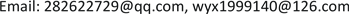Received: Apr. 5th, 2018; accepted: Apr. 19th, 2018; published: Apr. 26th, 2018ABSTRACT

Mechanical failures are mostly caused by the wear particles in the equipment. Detection of wear particles in the oil can effectively reflect the state of mechanical equipment. Online oil debris monitoring technique can effectively detect the concentration and the size of abrasive particle in the oil, thereby it can successfully monitor condition of mechanical equipment. When a particle passed through the sensor, the magnetic field of the sensor will be changed. An oil debris sensor with planar coil model has been established using ANSYS Maxwell software in this work. Two aspects of the transient and steady states of the model have been analyzed. Research on the influences of different parameters on the sensor in the steady state and the variation of abrasive passing through the sensor have been thoroughly conducted. The results of simulation have important meanings for the parameter optimization of oil debris sensor with planar coil.

Keywords:Oil Debris, Finite Element Method, Planar Coil, Sensor

1桂林电子科技大学，广西 桂林

2北京建筑大学，北京Copyright © 2018 by authors and Hans Publishers Inc.1. 引言

2. 平面线圈有限元分析理论基础

19世纪英国物理学家詹姆斯–麦克斯韦建立了一组描述电场、磁场与电荷密度、电流密度之间的偏微分方程，称之为麦克斯韦方程组。它由麦克斯韦-安培定律、法拉第电磁感应定律、高斯定律和高斯磁定律四个公式组成。

$\nabla ×H=i+\frac{\partial D}{\partial t}$ (1)

$\nabla ×E=-\frac{\partial B}{\partial t}$ (2)

$\nabla \cdot D=\rho$ (3)

$\nabla \cdot B=0$ (4)

$B=\int \text{d}B=\int \frac{{\mu }_{0}I}{\text{4π}}\cdot \frac{\text{d}l×r}{{r}^{3}}$ (5)

${B}_{x}=\frac{{\mu }_{0}I{R}_{a}z}{\text{4π}}{\int }_{0}^{\text{2π}}\frac{\mathrm{cos}\theta \text{d}\theta }{{\left[{\left(x-{R}_{a}\mathrm{cos}\theta \right)}^{2}+{\left(y-{R}_{a}\mathrm{sin}\theta \right)}^{2}+{z}^{2}\right]}^{3/2}}$ (6)Figure 1. Magnetic field distribution

${B}_{y}=\frac{{\mu }_{0}I{R}_{a}z}{\text{4π}}{\int }_{0}^{\text{2π}}\frac{\mathrm{sin}\theta \text{d}\theta }{{\left[{\left(x-{R}_{a}\mathrm{cos}\theta \right)}^{2}+{\left(y-{R}_{a}\mathrm{sin}\theta \right)}^{2}+{z}^{2}\right]}^{3/2}}$ (7)

${B}_{z}=\frac{{\mu }_{0}I}{\text{4π}}{\int }_{0}^{\text{2π}}\frac{{R}_{a}\left({R}_{a}-y\mathrm{cos}\theta -x\mathrm{cos}\theta \right)\text{d}\theta }{{\left[{\left(x-{R}_{a}\mathrm{cos}\theta \right)}^{2}+{\left(y-{R}_{a}\mathrm{sin}\theta \right)}^{2}+{z}^{2}\right]}^{3/2}}$ (8)

3. 平面线圈有限元分析分析

3.1. 稳态分析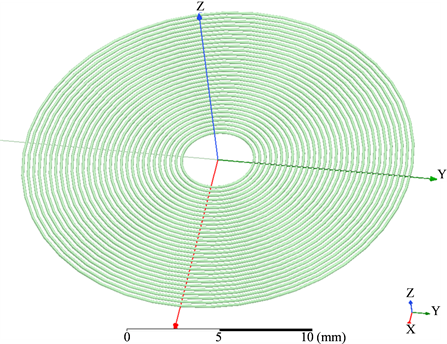Figure 2. Wear particle sensor 3-D model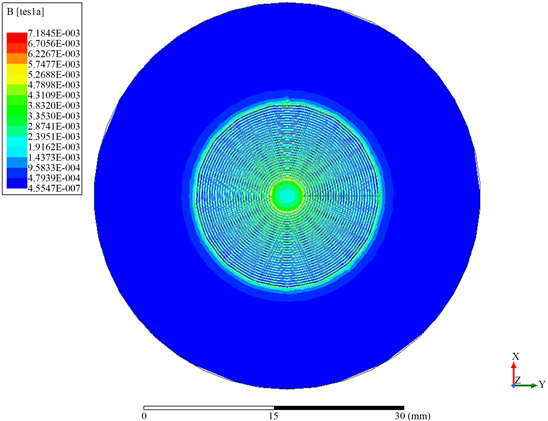(a)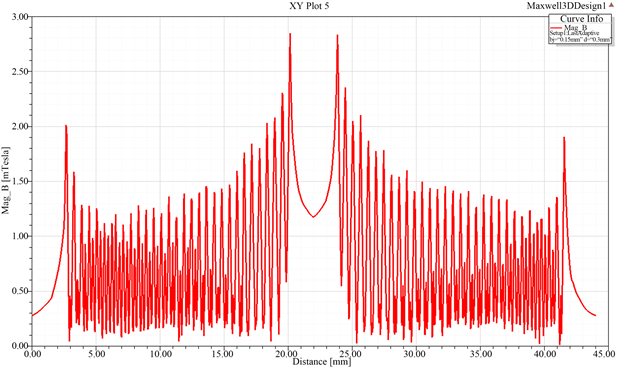(b)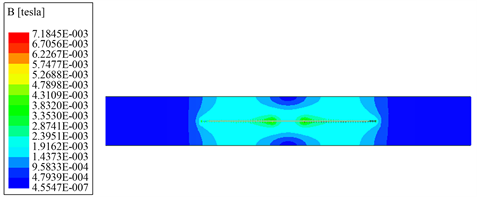(c)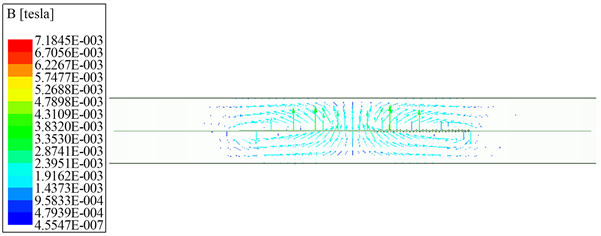(d)

Figure 3. Simulation result

3.2. 平面线圈瞬态分析

4. 结束语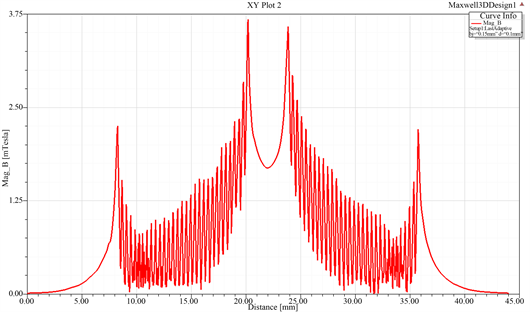(a)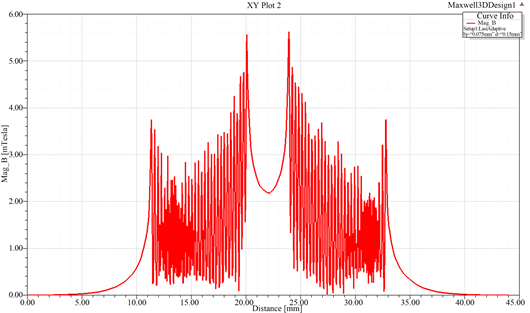(b)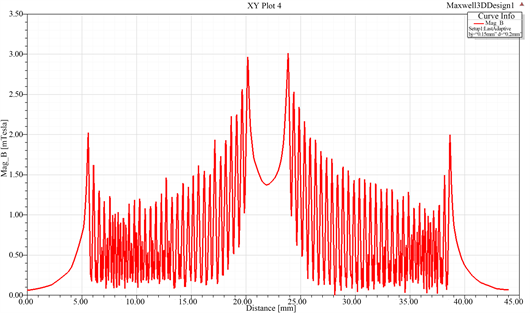(c)

Figure 4. Magnetic field distribution of planar coil at different parameters in YOZ plane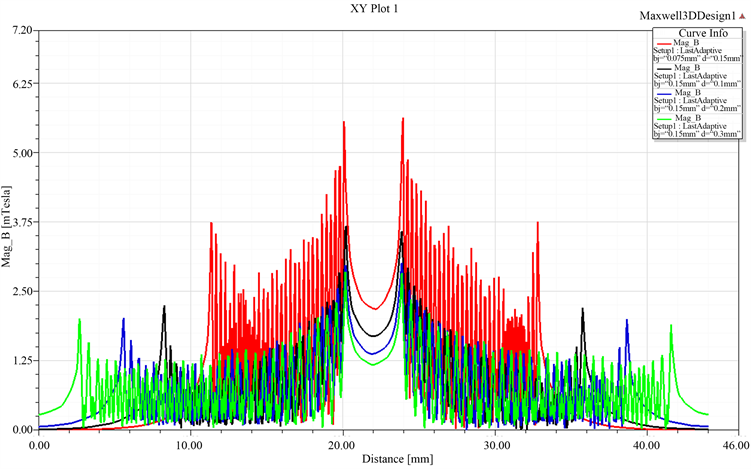Figure 5. Magnetic field distribution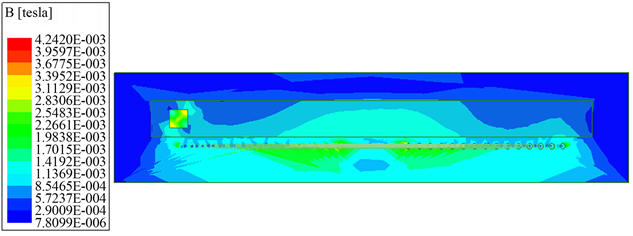(a)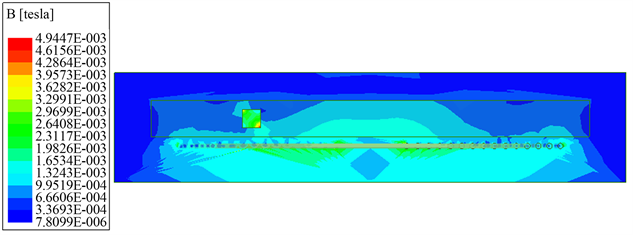(b)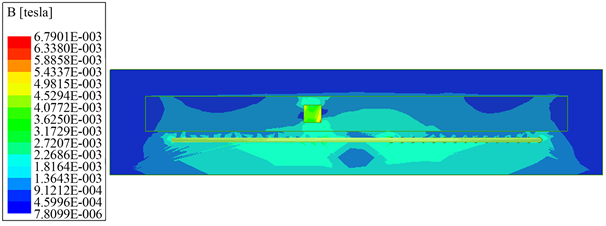(c)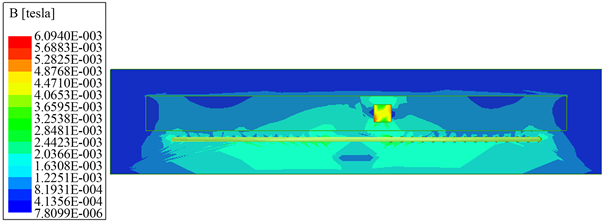(d)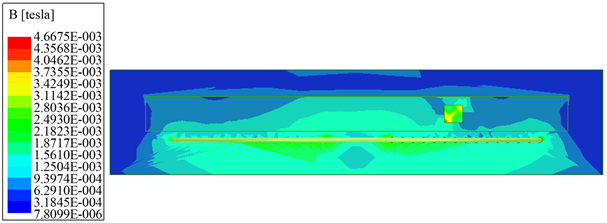(e)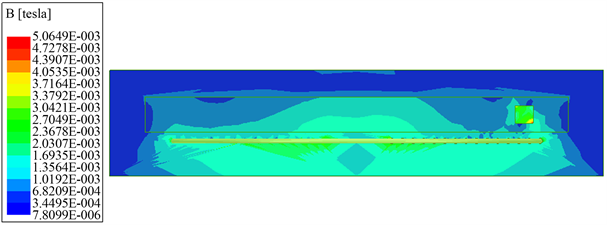(f)

Figure 6. Metal wear through the planar coil in magnetic flux density nephogram YOZ plane

Study on the Simulation of Planar Coil Oil Grinding Sensor[J]. 传感器技术与应用, 2018, 06(02): 58-67. https://doi.org/10.12677/JSTA.2018.62007

1. 1. Wu, C., Zheng, C. and Ma, B. (2011) Simulation Study on the Characteristic of Ferromagnetic Wear Debris in Inductive Wear Debris Sensor. Chinese Journal of Scientific Instrument, 32, 2774-2780.

2. 2. 谢友柏. 摩擦学面临的挑战及对策[J]. 中国机械工程, 1995(1): 6-9.

3. 3. 万耀青, 马彪. 滚动轴承的损伤机理与大颗粒磨损金属的状态监测[J]. 机械强度, 2000, 22(3): 161-193.

4. 4. 王衍学. 机械故障监测诊断的若干新方法及其应用研究[D]: [博士学位论文]. 西安: 西安交通大学, 2009.

5. 5. Ding, Y., Wang, Y. and Xiang, J. (2016) An Online Debris Sensor System with Vibration Resistance for Lubrication Analysis. Review of Scientific Instruments, 87, 025109. https://doi.org/10.1063/1.4941440

6. 6. Li, B., Yang, D., Hu, Z., et al. (2013) Theoretic Modeling and Numerical Simulation of the Electromagnetic Sensor for Online Wear Debris Monitoring. IEEE 2013 International Conference on Quality, Reliability, Risk, Maintenance, and Safety Engineering (QR2MSE), 15-18 July 2013, Chengdu, 1781-1784. https://doi.org/10.1109/QR2MSE.2013.6625922

7. 7. 范红波, 张英堂, 陶凤和. 铁磁质磨粒形态对电感式磨粒传感器输出特性的影响[J]. 传感技术学报, 2009, 22(10): 1402-1405.

8. 8. 张永祥, 明廷锋, 朴甲哲. 超声磨粒监测传感器设计研究[J]. 海军工程大学学报, 2002, 14(4): 28-31

9. 9. 殷勇辉. 光纤油液污染监测传感器设计[J]. 仪表技术与传感器, 2006(11): 3-4.

10. 10. 卞利, 李新星, 左洪福. 静电传感器在油液在线监测系统中的应用[J]. 润滑与密封, 2010, 35(3): 96-100.

11. 11. Du, L. and Zhe, J. (2011) A High Throughput Inductive Pulse Sensor for Online Oil Debris Monitoring. Tribology International, 44, 175-179. https://doi.org/10.1016/j.triboint.2010.10.022

12. 12. 于战果, 王宝光, 王海山. 应用探针式电容传感器检测发动机润滑油品质的研究[J]. 润滑与密封, 2007, 32(2): 171-173.